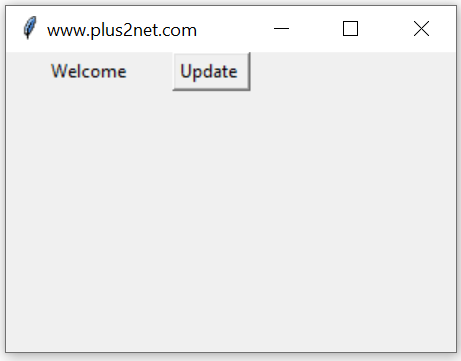# Python tkinter DoubleVar() trace

``e_v=tk.DoubleVar(master,value,name)``
`master`: (Optional)The variable is associated with, default value is parent window.
`value`:(Optional) We can set the initial value for the variable.
`name` : (Optional) Name given default is PY_VAR1

Tkinter DoubleVar() get(), set(), trace() methods to manage data and trigger call back functions

For an DoubleVar() so we can check the different modes of this variable, like this

w :Write- the variable is written by someone.
u :undefined – The variable is deletedHere is an example which uses w ( write ) mode to display the value of the variable when ever it changes.
We used one Button and used on Click event to change the value of this variable db1 from 5.1 to 10.34.
``b1 = tk.Button(my_w,text='Update',command=lambda:db1.set(10.34))``
This triggers the trace() which used the callback function my_r() to print the value of the variable ( 10.34 ) to our console.
``````import tkinter as tk
from tkinter import *
my_w = tk.Tk()
my_w.geometry("300x100")  # Size of the window

def my_r(*args):
print(db1.get()) #  Print when variable changes.

db1 = tk.DoubleVar(my_w) # declare DoubleVar()
db1.set(5.1) # assign value to variable

b1 = tk.Button(my_w,text='Update',command=lambda:db1.set(10.34))

db1.trace('w',my_r) # callback when data changes
my_w.mainloop()``````
Output
``10.34``
Use IntVar() for handling Integer data
Use DoubleVar() for handling Float Data

## set() and get() methods of DoubleVar

In above examples we used set() method to assign data to the Double variable and to read the data stored we used get() method. These two methods are frequently used in our scripts.
``````db1.set(10.34) # assign value to db1
print(db1.get()) # display the value assigned to db1``````

## Initializing DoubleVar

We can using set() method to assign data to DoubleVar after declaring or we can assign value while declaring the string variable.
``db1=tk.DoubleVar(value=5.1) # Assign value to db1``

## Length of DoubleVar

Before using len function, we have to convert the DoubleVar to string by using str()
``print(len(str(db1.get())))``

## Normal Variable and DoubleVar

DoubleVar() is a class in Tkinter. In a GUI application we require various events to be captured to trigger different functions (requirements). If we use normal Python variable with widgets then monitoring the changes is difficult. However by using a DoubleVar we can monitor the changes and trigger functions based on the requirements.

Example : Calculating division of two numbers or multiplication of user entered decimal numbers requires float variables. Here DoubleVar is used if you want to track the changes in the variable.

## Reading Entry box value using DoubleVarWe will connect the variable option of a Entry to BooleanVar() e_v and read the value.
By using trace() method of Double variable we will trigger a call back function.
Inside the function we will read the value of Double variable by using get() method and then update the text attribute of a Label by using config() method.
``````import tkinter as tk
my_w = tk.Tk()
my_w.geometry("400x200")  # Size of the window

e_v=tk.DoubleVar() # declare double varible
e_v.set(6.7) # assign the value to Double variable

e1=tk.Entry(my_w,textvariable=e_v,font=20)

l1=tk.Label(my_w,text='Output',font=22,bg='yellow')
def my_upd(*ags): # call back function
my_data=e_v.get() # reading value of Double var
l1.config(text=str(my_data)) # display in Label

e_v.trace('w',my_upd) # track the changes of Double variable
my_upd() # call the function to update the display
my_w.mainloop()  # Keep the window open``````

## Application using DoubleVar

For conversion of measurement units like feet to meter and from meter to feet we used DoubleVar.

Subscribe to our YouTube Channel here

## Subscribe

* indicates required
Subscribe to plus2netplus2net.com